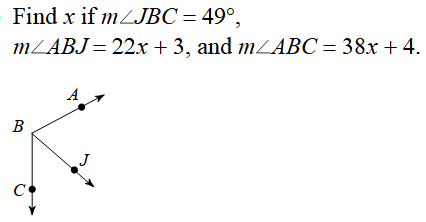chapter 1 Skills Maint 4
If <PQT = 88, What is the measure of <SQT? *
1 point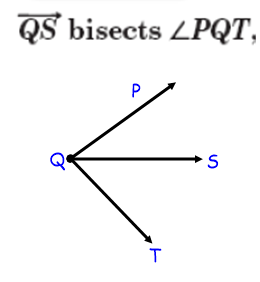Define a Ray *
1 point
Find x. L + L = B *
1 point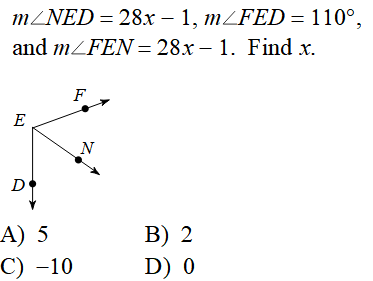Identify the INCORRECT way to name this angle *
1 point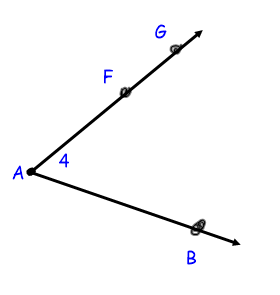L + L = B find x *
1 point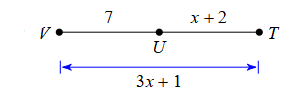What is the measure of <CGD? *
1 point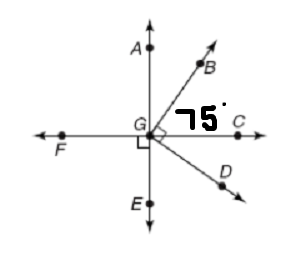READ CAREFULLY. FIND X If <PQT = 12x - 12, and <PQS = 5x + 16, *
1 pointFind x *
1 point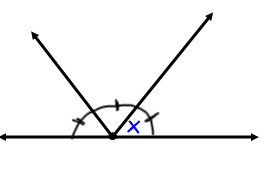Which angle is adjacent to <KFG *
1 point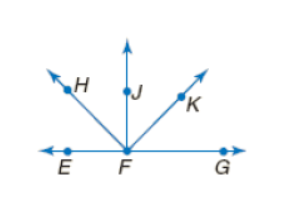Find x *
1 point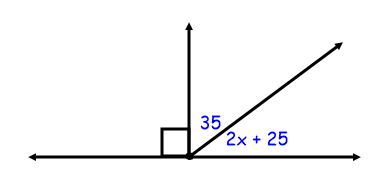Find x. *
1 point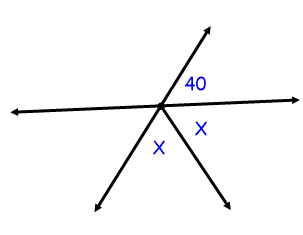Find x. *
1 point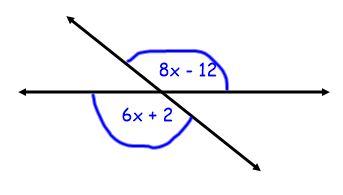period *
*
1 point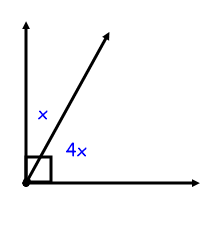Last Name *
L + L = B find x *
1 point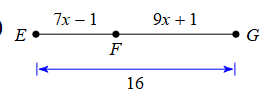Find x. L + L = B *
1 point